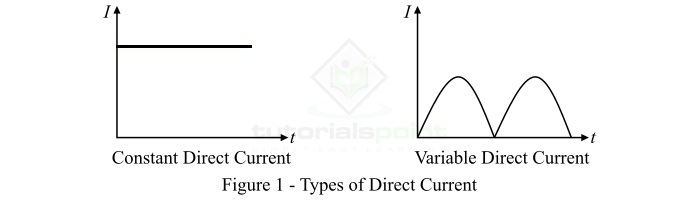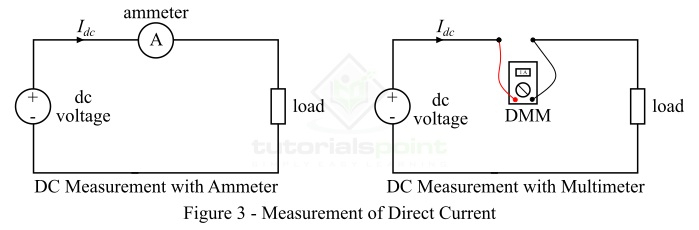# What is Direct Current? – Definition, History, Measurement, and Applications

In electrical and electronics, the term electric current (or current) is used to represent the flow of electric charge (electrons or holes) in a particular direction. Hence, the electric current is simply defined as the directed flow of electrons (in metals), and electrons and holes (in semiconductors). In actual practice, the electric current can only flow through metals and semiconductors, while insulators block the flow of electric current through them. Based on the direction of flow, the electrical current is broadly classified into two types namely direct current (DC) and alternating current (AC).

In this article, we will discuss the definition, symbol, formula, history, measurement, and applications of direct current (DC). So letŌĆÖs begin with the definition of direct current.

## What is Direct Current?

Direct Current, abbreviated as DC, is defined as the unidirectional flow of electric charge (mainly electrons) through a conductor. Therefore, the type of electric current which flows only in one direction through the conductor is called DC or direct current. Sometimes, we use the term DC Current to refer to the direct current. The direct current can flow through all the conducting materials like metallic wires or semiconductors.

The flow of direct current through a material is caused by the direct voltage. The direct voltage is created by a source of DC power like a cell, battery, DC generator, etc. When the direct voltage is applied across the two ends of a conductor, it forces the free electrons to flow through the conductor in a particular direction constituting the direct current through the conductor. The direction of the flow of electrons is from the negative terminal to the positive terminal of the source, while the direction of direct current is opposite to that of electrons, i.e. from the positive terminal to the negative terminal of the source of direct voltage.

### Actual Meaning of Direct Current

In electrical and electronics, the term "direct" is used to denote only one electrical polarity of current or voltage. For example, a battery always has fixed positive and negative terminals and they do not change with time. Thus, the battery is an example of source of DC power (direct voltage and current).

## Types of Direct Current

As we know, the electrical polarity of the direct current always remains constant, but the magnitude of the direct current may change. Thus, based on the variation in the magnitude of direct current, it can be classified into the following two types ŌłÆ

• Constant Direct Current (Constant DC) ŌłÆ The type of direct current whose magnitude remain constant all the time is known as constant direct current. The constant direct current is also referred to as time-invariant current. The constant direct current is usually represented by the symbol "I". Examples of constant DC are the current obtained from a cell, battery, or a DC generator.

• Variable Direct Current (Variable DC) ŌłÆ The direct current whose magnitude changes with time is referred to as variable direct current. It is also known as timevarying current as its magnitude changes with time. We use the symbol "i" to represent the variable DC. Examples of variable DC is the raw output of a rectifier, or a fluctuating voice signal on a telephone line.## History of Direct Current

The following points give a historical view of the production of direct current ŌłÆ

• The credit of discovery of the direct current goes to an Italian physicist Alessandro Volta, who developed a Voltaic pile (first electric battery) in 1800.

• The nature of how the direct current flows was told by a French physicist Andre-Marie Ampere who supposed that the direct current flows in one direction from positive to negative.

• In 1882, American Inventor Thomas Edison launched his incandescent light bulb to work on low voltage direct current for indoor electric lighting in homes and offices.

• In 1950s, the high voltage direct current transmission system was developed which is now being used for long distance high voltage transmission of electric power.

## Direct Current Circuits

An electric circuit flowed by direct current (DC) is referred to as a direct current circuit. A DC circuit is usually a combination of constant voltage sources, constant current sources, and resistors.In DC circuits, the value of current is independent of time. Also, the direct current does not depend on the past history of the circuit. Therefore, the mathematical equations that represent a DC circuit do not have integrals or derivatives with respect to time. In electronics, a DC circuit is one that is powered by a DC voltage source like a cell or battery.

## How to Measure Direct Current?

The direct current in an electric circuit can be measured by using either a DC ammeter or a multimeter.

• DC Measurement using Ammeter ŌłÆ A DC ammeter is connected in series with the circuit whose current is to be measure. The deflection of pointer on the scale of ammeter or the digital display shows the value of direct current flowing through the circuit.

• DC Measurement using Multimeter ŌłÆ The positive (red) and negative (black) probes of the multimeter are connected in the circuit as shown in Figure-3. The knob of the multimeter is then set to the direct current measurement. The reading of the direct current is shown on the digital screen of the multimeter.## Applications of Direct Current

The following are the major applications of Direct Current ŌłÆ

• Direct current is required for the operation of most electronic circuits like toys, smartphones, laptops, etc.

• Direct current is used for emergency lighting in buildings.

• Direct current is used in automotive applications.

• Electric vehicles use direct current to run.

• Direct current is also used in telecommunication systems.

• Direct current is used for long distance high voltage power transmission in HVDC systems.

• Direct is also used in light aircraft electrical systems.

## Conclusion

To conclude, direct current is one which has a constant electrical polarity, i.e. flow only in one direction. For this reason, the direct current is also called a unidirectional current. The electric circuit followed by a direct current is referred to as a DC circuit. The direct current is used in several applications such as HVDC transmission, computers and laptops, smartphones, electric vehicles, etc.